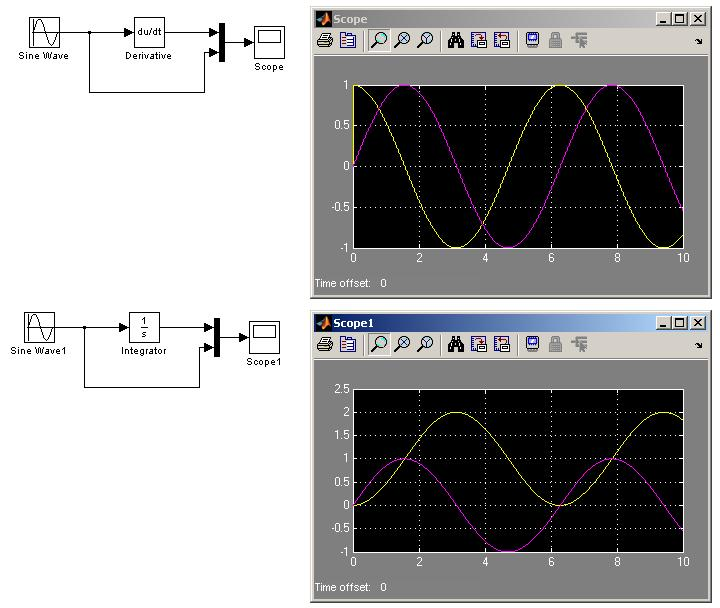# Derivation and Integration in MATLAB/SIMULINK

Status
Not open for further replies.

#### powersysderive matlab

I try to implement derivator and integrator in MATLAB/SIMULINK as shown in Figure below. The input to the derivator or integrator is a sinewave. I found out an offset at the output of the integrator. How to remove the offset? The model uses default settings.#### mowgli

##### Full Member level 2matlab derive

Hello powersys,

what you see is due to the fact that mathematically the result of the integration is the calculation of the area (with sign) enclosed by the input curve: in your example the sin input starts with zero phase so the integral initially accumulates positive area for a half period then it decreases to 0 by subtracting the negative area enclosed by the sin wave in the following half period and so on.
If you start the sin with Π/2 offset you should obtain a balanced output from the integrator.

Regards
Mowgli

### powersys

Points: 2

#### powersysderivation in matlab

mowgli said:
what you see is due to the fact that mathematically the result of the integration is the calculation of the area (with sign) enclosed by the input curve: in your example the sin input starts with zero phase so the integral initially accumulates positive area for a half period then it decreases to 0 by subtracting the negative area enclosed by the sin wave in the following half period and so on.
If you start the sin with Π/2 offset you should obtain a balanced output from the integrator.